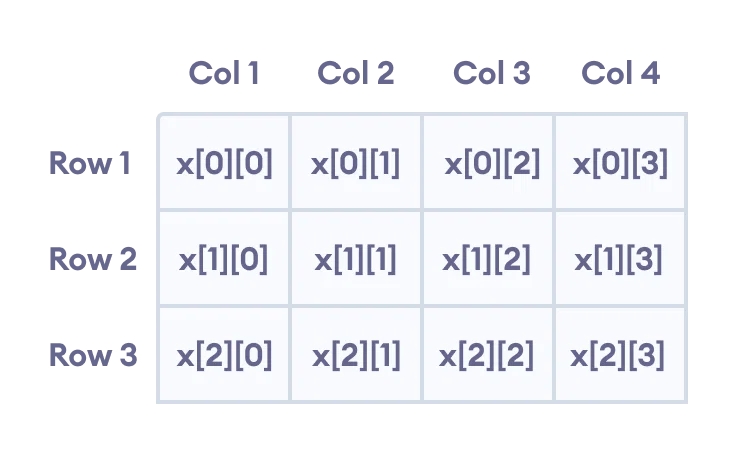# C++ arrayLike other programming languages, array in C++ is a group of similar types of elements that have contiguous memory location.

In C++ std::array is a container that encapsulates fixed-size arrays. In C++, the array index starts from 0. We can store only a fixed set of elements in a C++ array.

## Types of array in c++

There are 2 types of arrays in C++ programming:

1. Single Dimensional Array
2. Multidimensional Array

## C++ Single Dimensional Array

An array of one dimension is known as a onedimensional array or 1-D array or single-dimensional array.

Syntax:

`array_name[array_size]`

Example:

```#include <iostream>
using namespace std;
int main()
{
int arr={10, 0, 20, 0, 30};  //creating and initializing array
//traversing array
for (int i = 0; i < 5; i++)
{
cout<<arr[i]<<"\n";
}
}  ```

Output:

```10
0
20
0
30```

## C++ MultiDimensional Array

An array of two-dimension is known as a twodimensional array or 2-D array or multidimensional array. for example

`int x;`

Here, x is a two-dimensional array. It can hold a maximum of 12 elements.

We can think of this array as a table with 3 rows and each row has 4 columns as shown below.Elements in two-dimensional array in C++ Programming

Example for Two Dimensional Array

```// C++ Program to display all elements
// of an initialised two dimensional array

#include <iostream>
using namespace std;

int main() {
int test = {{2, -5},
{4, 0},
{9, 1}};

// use of nested for loop
// access rows of the array
for (int i = 0; i < 3; ++i) {

// access columns of the array
for (int j = 0; j < 2; ++j) {
cout << "test[" << i << "][" << j << "] = " << test[i][j] << endl;
}
}

return 0;
}```

Output

```test = 2
test = -5
test = 4
test = 0
test = 9
test = 1```

## Advantages of C++ Array

• Code Optimization (less code)
• Random Access
• Easy to traverse data
• Easy to manipulate data
• Easy to sort data etc.

## Disadvantages of C++ Array

• Fixed size
5 1 vote
Article Rating

Learn C++ App available on play store Download Now.
Do you want to hire us for your Project Work? Then Contact US.